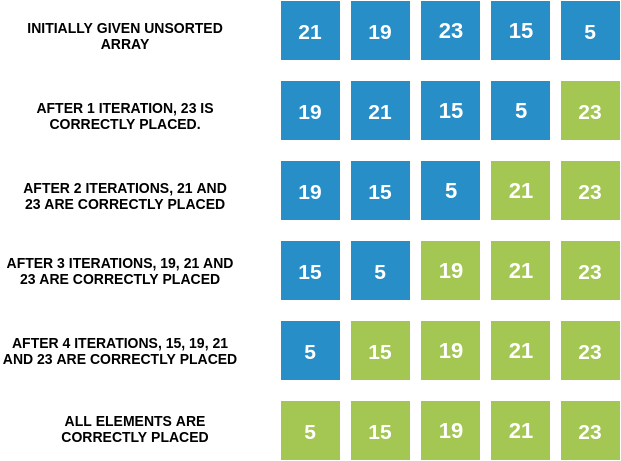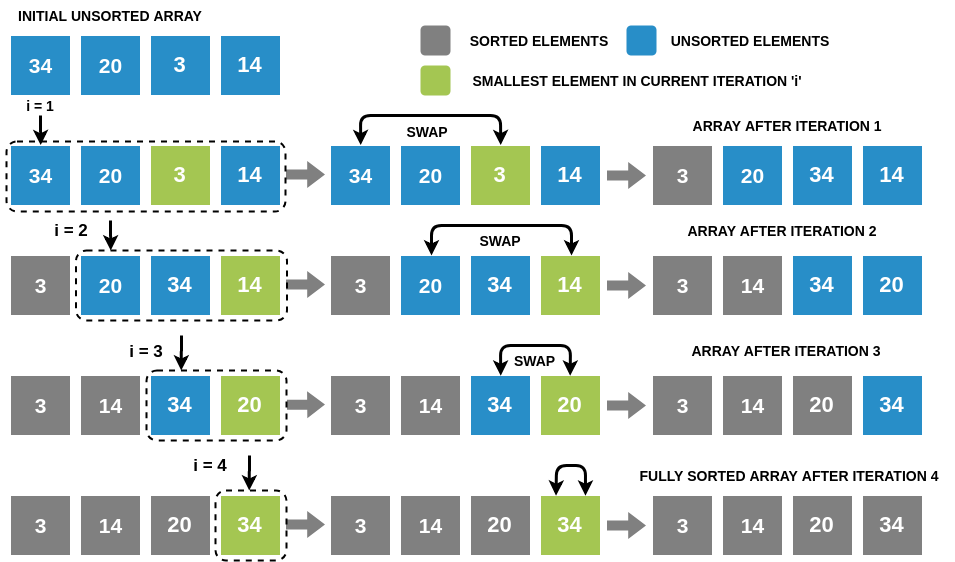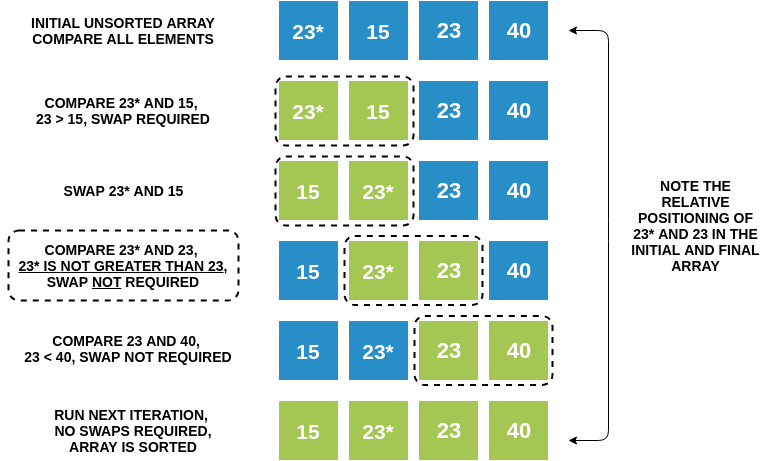×

Search anything:

# Bubble sort vs Selection sort

#### Sorting Algorithms AlgorithmsGet this book -> Problems on Array: For Interviews and Competitive Programming

Bubble sort and Selection sort are both comparison-based sorting algorithms which can be differentiated through the methods they use for sorting. But before going through the key differences between them, let's understand how both of these algorithms work.

1. Basics of Bubble Sort
2. Basics of Selection Sort
3. Key Differences between Bubble Sort and Selection Sort
4. Summary
6. Conclusion

## Basics of Bubble Sort

Bubble sort is a comparison-based sorting algorithm that involves making passes over the input array where, in each pass, adjacent elements are swapped if they are not present in the required order (ascending or descending). It keeps on making the passes until the whole array is sorted.

### Bubble Sort Algorithm (in ascending order)

1. Starting from the first index, compare the first two elements. If the first element is greater than the second element, swap them; else, leave them as it is.
2. Now, compare the next two elements. Swap them if they are not in order. This process goes on until the last element.

The first pass is complete — largest element present will be at the end of the array.

1. The same process goes on for the remaining passes. After each pass, the largest element among the unsorted elements is placed at the end.

In each pass, the comparison takes place up to the last unsorted element. The array is sorted when all the unsorted elements are placed at their respective correct positions.

Optimized Bubble Sort: If, in a pass, we do not make any swaps, then we don’t need to make any further passes.

### Iteration by Iteration Visualization of Bubble Sort### Bubble Sort Code in Java

``````public static void bubbleSort(int arr[])
{
int n = arr.length;
for (int i = 0; i < n - 1; i++)
for (int j = 0; j < n - i - 1; j++)
if (arr[j] > arr[j + 1]) {
// swap arr[j+1] and arr[j]
int temp = arr[j];
arr[j] = arr[j + 1];
arr[j + 1] = temp;
}
}
``````

## Basics of Selection Sort

Selection sort is also a comparison-based sorting algorithm that involves dividing the given array into two parts:

• Sorted sub-array, which is initially empty
• Unsorted sub-array, which initially contains all the elements

Considering we want to sort the array in ascending order:
In every iteration, we pick the minimum element from the unsorted sub-array and place it at the end of the sorted sub-array. We repeat this entire process until the unsorted array becomes empty.

### Selection Sort Algorithm (in ascending order)

Repeatedly select the smallest element from the unsorted sub-array and swap it to its proper index.

1. Find the smallest item in the array, and swap it with the first item.
2. Find the next smallest item in the array, and swap it with the next item.
3. Continue until all items in the array are sorted.

### Iteration by Iteration Visualization of Selection Sort### Selection Sort Code in Java

``````    void selectionSort(int arr[])
{
int n = arr.length;

for (int i = 0; i < n-1; i++)
{
// Finding the minimum element in each loop
int min_idx = i;
for (int j = i+1; j < n; j++)
if (arr[j] < arr[min_idx])
min_idx = j;

// Putting the minimum element at correct position
int temp = arr[min_idx];
arr[min_idx] = arr[i];
arr[i] = temp;
}
}
``````

## Key Differences between Bubble Sort & Selection Sort

Below are the parameters used to judge the differences between the two algorithms:

### Functionality

Bubble sort compares the adjacent elements and swap them accordingly while selection sort selects the minimum element from the unsorted sub-array and places it at the next position of the sorted sub-array.

### Efficiency

Another major difference between bubble sort and selection sort is that selection sort is efficient compared to bubble sort.

### Speed

Selection sort is faster compared to bubble sort as it requires lesser number of comparisons.

### Method

One other difference between bubble sort and selection sort is that the bubble sort uses item exchanging method while selection sort uses item selection method.

### Stability

Bubble sort is a stable algorithm in which relative order between equal elements will be maintained. Selection sort is an unstable algorithm.

##### Stability of Bubble Sort### Iterativeness

Selection sort is a non-iterative algorithm, while bubble sort is an iterative algorithm.

### Descending Order

• Selection sort algorithm can sort the given elements in the list either in ascending order or descending order.
• Bubble sort algorithm sorts the given elements in the list in ascending order and afterwards the list is reversed so as to print the elements of the array in descending order.

### Time Complexity

The time complexity of both the algorithms is as follows:

##### Best Case:
• Selection sort: The best-case complexity is O(N^2) as to find the minimum element at every iteration, we will have to traverse the entire unsorted array.
• Bubble sort: The best-case complexity is O(N). It happens when we have an already sorted array. We will have to run one iteration (N-1 comparisons) to determine this. Time complexity will be O(N) in this case.
##### Worst Case:
• Selection sort: The worst-case complexity is O(N^2) as we will have to traverse the entire unsorted array to find the minimum element at every iteration.
• Bubble sort: The worst-case complexity is O(N^2). It happens when we have a reverse sorted array, and in that case, we will have to make (N-1) passes, where N is the number of elements present in the array.
##### Average Case:

For random datasets, both algorithms take O(N^2) time complexity.

### Applications

• Selection sort can sort a linked list as we can efficiently remove the smallest element and append it in the sorted list.
• Bubble sort is used to detect minor errors in a sorted dataset and sort an almost sorted dataset quickly.

## Summary: Bubble vs Selection Sort

The main difference between bubble sort and selection sort is that bubble sort operates by repeatedly swapping the adjacent elements if they are in the wrong order. In contrast, selection sort sorts an array by repeatedly finding the minimum element (or, maximum element if we we want to sort the array in descending order) from the unsorted part and placing that at the beginning of the array.

Parameter Bubble Sort Selection Sort
and swapped
Largest/Smallest element is selected
and swapped with the last element
(in case of ascending/descending order).
Efficiency Less Efficient More Efficient
Speed Slower Faster
No. of comparisons Greater Lesser
Method Exchanging Selection
Stable Yes No
In-place Yes Yes
Iterativeness Iterative algorithm Non-iterative algorithm
Descending Order Array is first sorted in
ascending order and then reversed
to display the elements
in descending order.
Can sort the given elements
in the array either in ascending
order or descending order.
Best-case Time Complexity O(N) O(N^2)
Worst-case Time Complexity O(N^2) O(N^2)
Average-case Time Complexity O(N^2) O(N^2)
Space Complexity O(1) O(1)
errors in the almost
sorted dataset
Can be applied on
sorting algorithm
Effective when swap operations
are costly
large data sets
Not scalable

## Question 1

#### The given array is arr = {1, 2, 4, 3}. Bubble sort is used to sort the array elements. How many iterations will be done to sort the array?

4
2
1
0
Even though the first two elements are already sorted, bubble sort needs 4 iterations to sort the given array. 8. How can you improve the best case efficiency in bubble sort? (The input is already sorted)

## Question 2

#### What will be the space complexity of the bubble sort algorithm?

O(N)
O(2^N)
O(1)
O(N^2)
No extra space/memory is used besides that already used to store the original array and the temporary variable used to swap array elements. The sorting is directly done on the original array. This notation is used to specify constant space complexity.

## Question 3

#### What is the average case complexity of selection sort?

O(N^2)
O(NlogN)
O(logN)
O(N)
In the average case, even if the input is partially sorted, selection sort behaves as if the entire array is not sorted. Selection sort is insensitive to input.

## Question 4

#### What is the disadvantage of selection sort?

It is not scalable
It requires auxiliary memory
It can be used for small keys
It takes linear time to sort the elements
As the input size increases, the performance of selection sort decreases.

## Question 5

#### The given array is arr = {3,4,5,2,1}. Find the number of iterations in bubble sort and selection sort respectively.

5 and 4
4 and 5
2 and 4
2 and 5
Since the input array is not sorted, bubble sort takes 5 iterations and selection sort takes 4(n-1) iterations.

## Conclusion

In this article at OpenGenus, we have learned the concept of sorting using selection sort and bubble sort and understood the key differences between selection sort and bubble sort like methods used for sorting, their efficiencies, stabilities, functionalities, time complexities, speed, applications, etc.